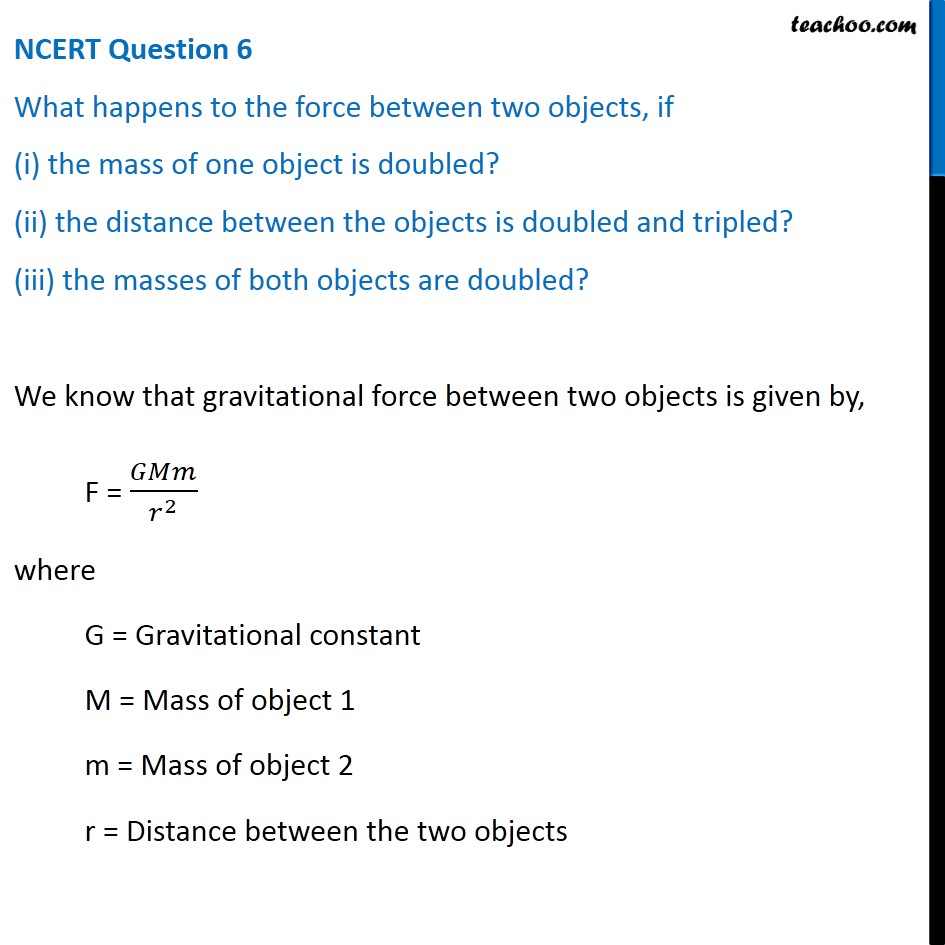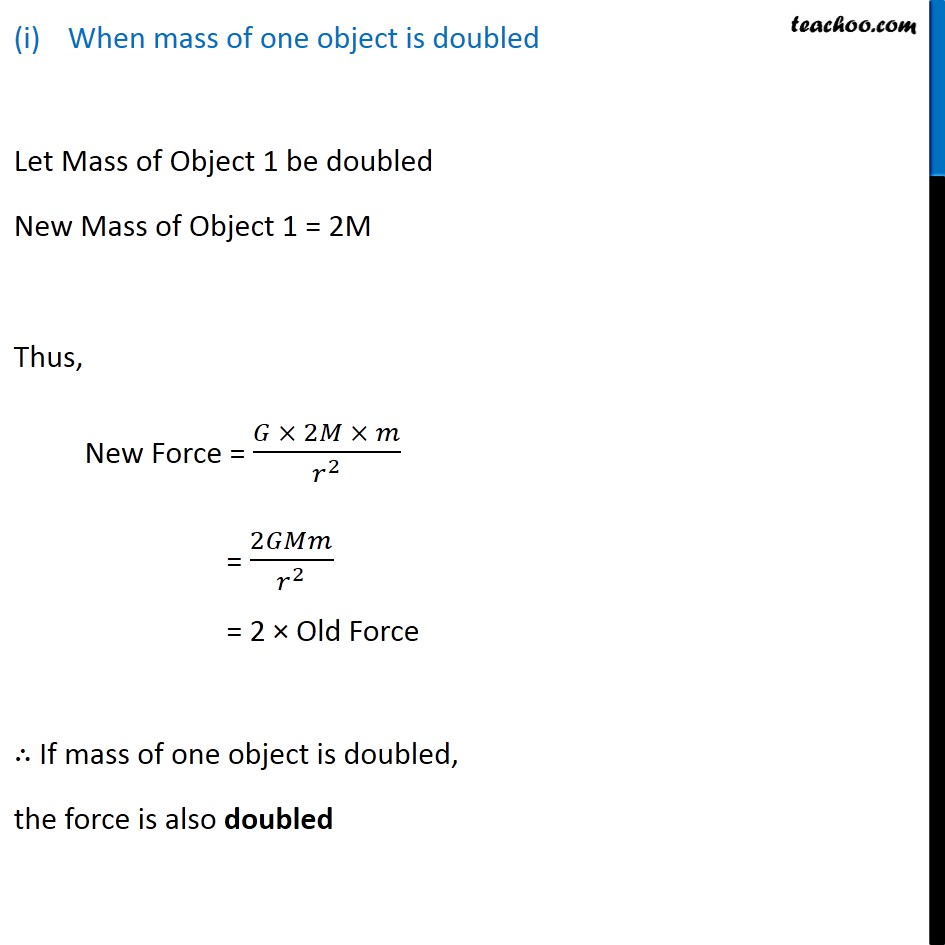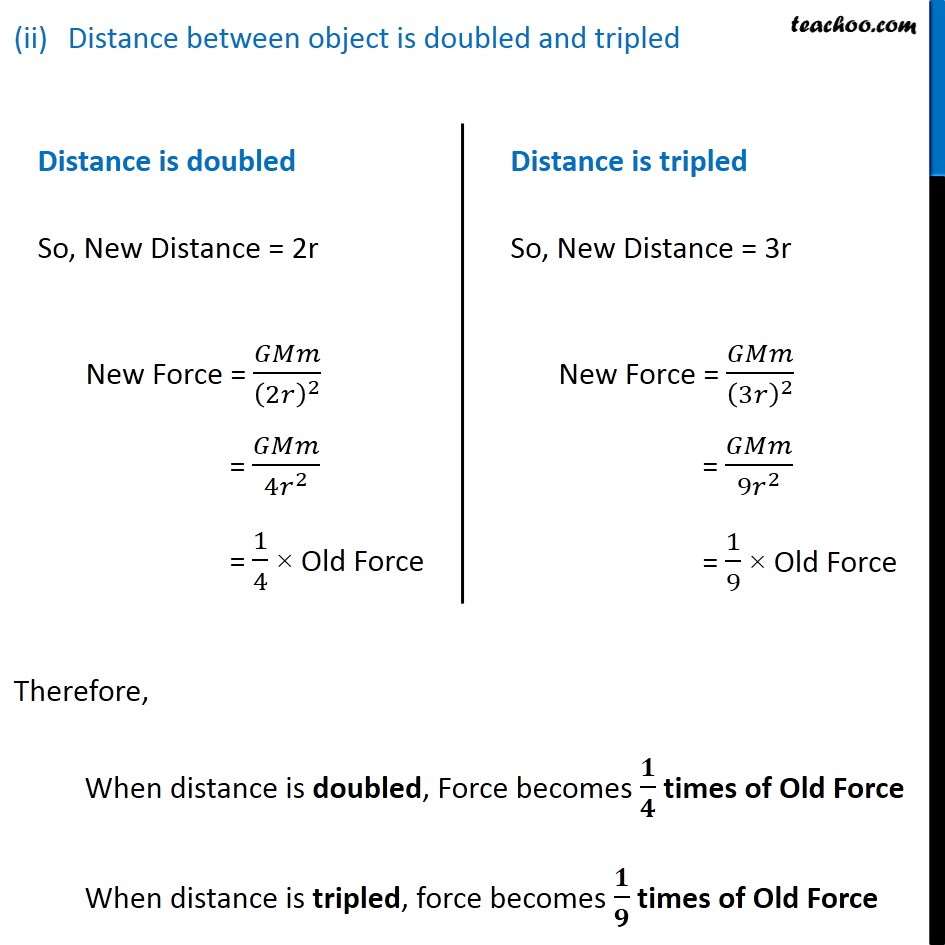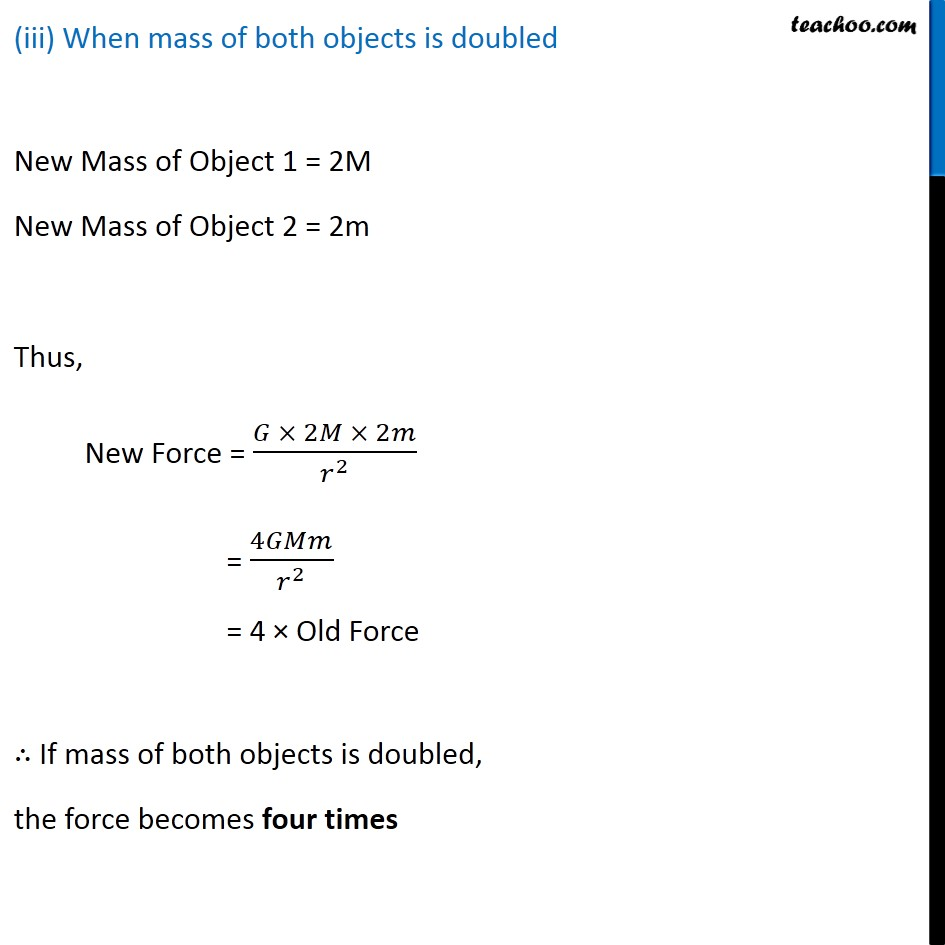NCERT Questions

Class 9
Chapter 10 Class 9 - GravitationLearn in your speed, with individual attention - Teachoo Maths 1-on-1 Class

### Transcript

NCERT Question 6 What happens to the force between two objects, if (i) the mass of one object is doubled? (ii) the distance between the objects is doubled and tripled? (iii) the masses of both objects are doubled? We know that gravitational force between two objects is given by, F = 𝐺𝑀𝑚/𝑟^2 where G = Gravitational constant M = Mass of object 1 m = Mass of object 2 r = Distance between the two objects When mass of one object is doubled Let Mass of Object 1 be doubled New Mass of Object 1 = 2M Thus, New Force = (𝐺 × 2𝑀 × 𝑚)/𝑟^2 = 2𝐺𝑀𝑚/𝑟^2 = 2 × Old Force ∴ If mass of one object is doubled, the force is also doubled Distance between object is doubled and tripled Distance is doubled So, New Distance = 2r New Force = 𝐺𝑀𝑚/(2𝑟)^2 = 𝐺𝑀𝑚/(4𝑟^2 ) = 1/4 × Old Force Distance is tripled So, New Distance = 3r New Force = 𝐺𝑀𝑚/(3𝑟)^2 = 𝐺𝑀𝑚/(9𝑟^2 ) = 1/9 × Old Force Therefore, When distance is doubled, Force becomes 𝟏/𝟒 times of Old Force When distance is tripled, force becomes 𝟏/𝟗 times of Old Force (iii) When mass of both objects is doubled New Mass of Object 1 = 2M New Mass of Object 2 = 2m Thus, New Force = (𝐺 × 2𝑀 × 2𝑚)/𝑟^2 = 4𝐺𝑀𝑚/𝑟^2 = 4 × Old Force ∴ If mass of both objects is doubled, the force becomes four times